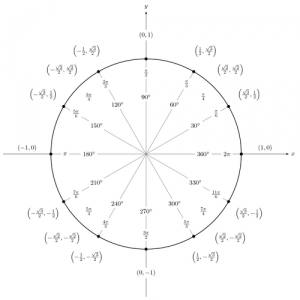# Are Diameter and Circumference the Same

The answer to the question “are diameter and circumference the same” is they are not. The circumference refers to the distance around a circle. The diameter is the distance along a circle through its middle.

### How to Calculate the Diameter of a CircleGet a ruler and put it at the circle’s edge. The next step is extending the ruler along the circle’s center point up to the opposing end. Take note of where the ruler is at the circle’s opposing edge. This will be directly from the beginning point. The result is the circle diameter.

### How to Calculate Circle Circumference

The first step is to get the circle diameter using the method described earlier. Next, get the circumference formula, which is C = pi * d. The d is the diameter and c is the circumference. The pi can be 22/7 or 3.14. 3.14 is used more often. To get the circumference, double the radius. This is done via C = pi * 2r. R is the radius.

If you are having trouble visualizing, think of the diameter as a line slicing a circle exactly in half. Radius refers to the circle’s center to any point in the shape. In other words, it is half the circle diameter. The pi is the circumference ratio in relation to the diameter. Known as a constant, pi is an unchanging number. This number remains the same whether the circle is small or large. Pi is usually known as 3. Needless to say, the measurements will only be valid if similar units are used.

### How to Get a Radius and Area from Circumference

The first step is to divide the circumference by pi. Divide the result by pi in half to get the radius. Next you have to get the radius squared. Multiply this by pi and you will get the area.

### Other Interesting Facts

A circle diameter is a circle’s longest chord. The word is Greek in origin meaning across. These days, diameter length is also known as a diameter. If speaking about a convex shape, the diameter is known as the largest distance between lines that are opposite. The smallest distance is the width.

As you can see the answer to the question are diameter and circumference the same is simple. They are not the same. But they are related. Knowing how to calculate these sizes will be key to understanding a circle’s properties.